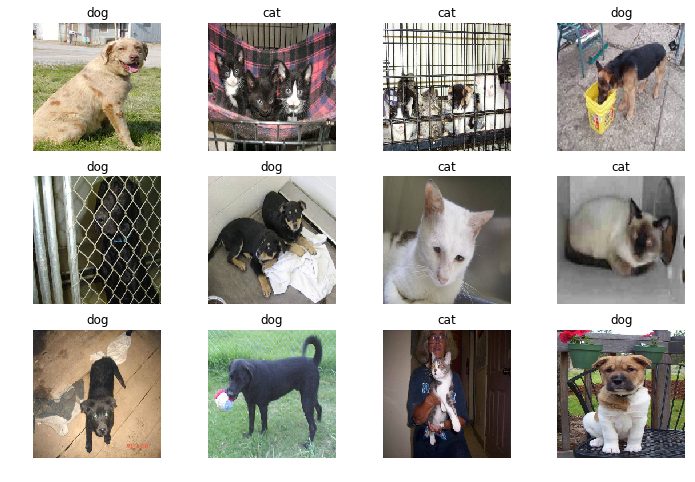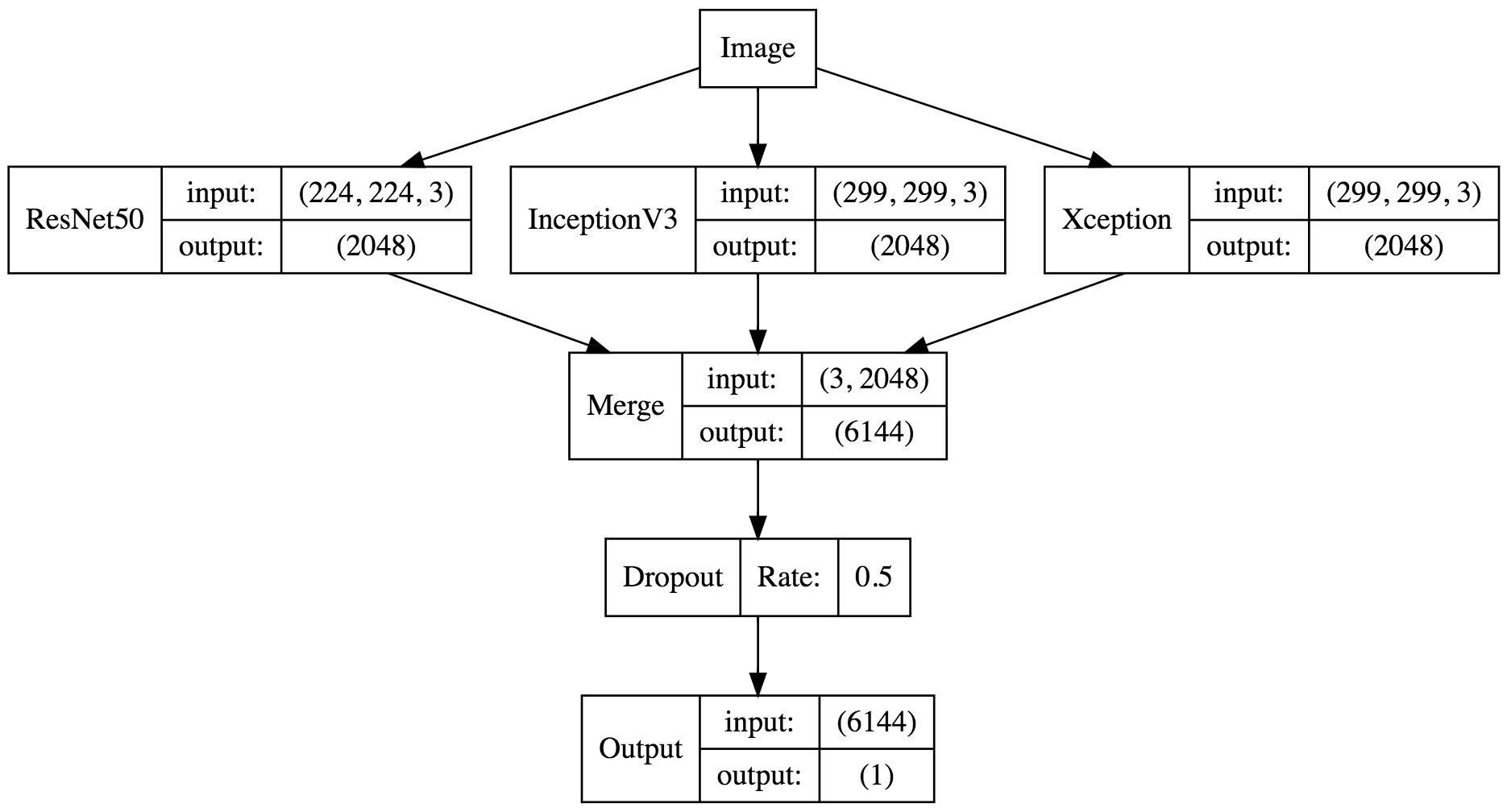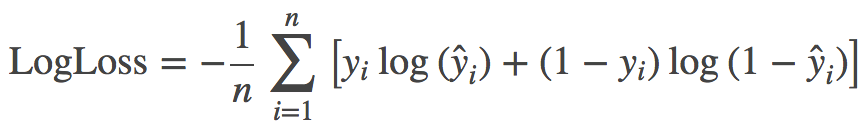# 猫狗大战# 导出特征向量

• train (25000, 2048)
• test (12500, 2048)
• label (25000,)

# 载入特征向量

• gap_ResNet50.h5
• gap_InceptionV3.h5
• gap_Xception.h5

# 构建模型# 预测测试集

$$\textrm{LogLoss} = - \frac{1}{n} \sum_{i=1}^n \left[ y_i \log(\hat{y}_i) + (1 - y_i) \log(1 - \hat{y}_i)\right]$$